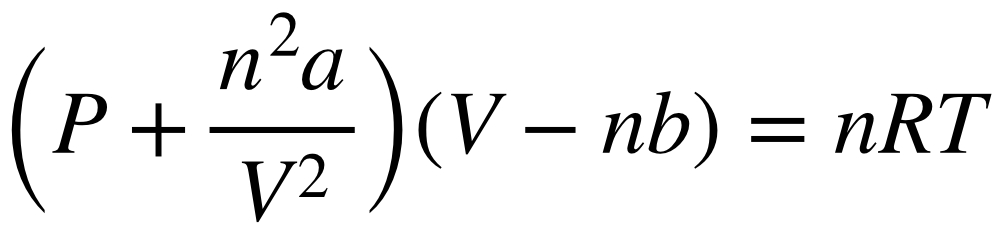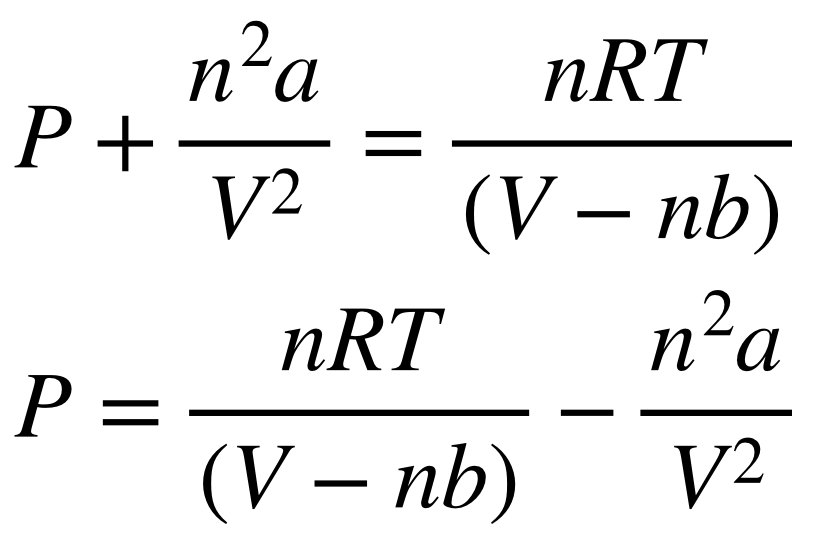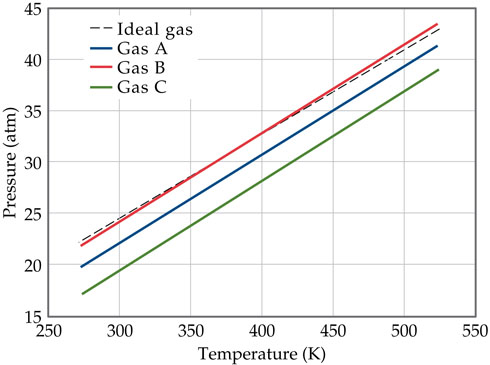# Problem: The graph below shows the change in pressure as the temperature increases for a 1 mol sample of a gas confined to a 1 L container. The four plots correspond to an ideal gas and three real gases: CO2, N2, and Cl2.At room temperature, all three real gases have a pressure less than the ideal gas. Which van der Waals constant, a or b, accounts for the influence intermolecular forces have in lowering the pressure of a real gas?

###### FREE Expert Solution

The Van der Waals' Equation is as follows:where a is the polarity coefficient, which accounts for the attractive or repulsive interactions of the gas molecules, and b is the size coefficient, which accounts for the size of the individual gas molecules. This equation show how real gases deviate from ideal behavior.

We can rearrange this equation to see how both a and b affect the pressure of the gas.100% (97 ratings)###### Problem Details

The graph below shows the change in pressure as the temperature increases for a 1 mol sample of a gas confined to a 1 L container. The four plots correspond to an ideal gas and three real gases: CO2, N2, and Cl2.At room temperature, all three real gases have a pressure less than the ideal gas. Which van der Waals constant, a or b, accounts for the influence intermolecular forces have in lowering the pressure of a real gas?